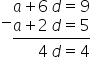Mathematics
Easy

Question

# Determine the first term and common difference whose 3rd term is 5 and the 7th term is 9

## 3 , 14 , 2 5 , 31 , 3Hint:

## The correct answer is: 3 , 1

### The first term of the progression is denoted by "a"The common difference is "d".The third term is a3 = 5The fifth term is a7 = 9Common difference is the fixed difference between the consecutive numbers of the sequence. We have to add the common difference to the preceding term, to get the next term. It can be negative or positive number. It can also have value zero.The formula for nth term of a arithmetic progression is given as follows:an = a + (n – 1)dLet’s see the formula for fifth and seventh term.a3= a + (3 – 1)d5 = a + 2d               …(1)a7 = a + (7 – 1)d9 = a + 6d               …(2)Solving the equations (1) and (2). We will subtract (1) from (2).4d = 4So, d = 1We will substitute the value of d in equation (1).5 = a + 2(1) 5 = a + 2a = 3So, the first term of the sequence is 3 and the common difference is 1.The right option is 1,3.

For such questions, we should know the formula to find any number of the terms.

### Related Questions to study#### With Turito Foundation.#### Get an Expert Advice From Turito.